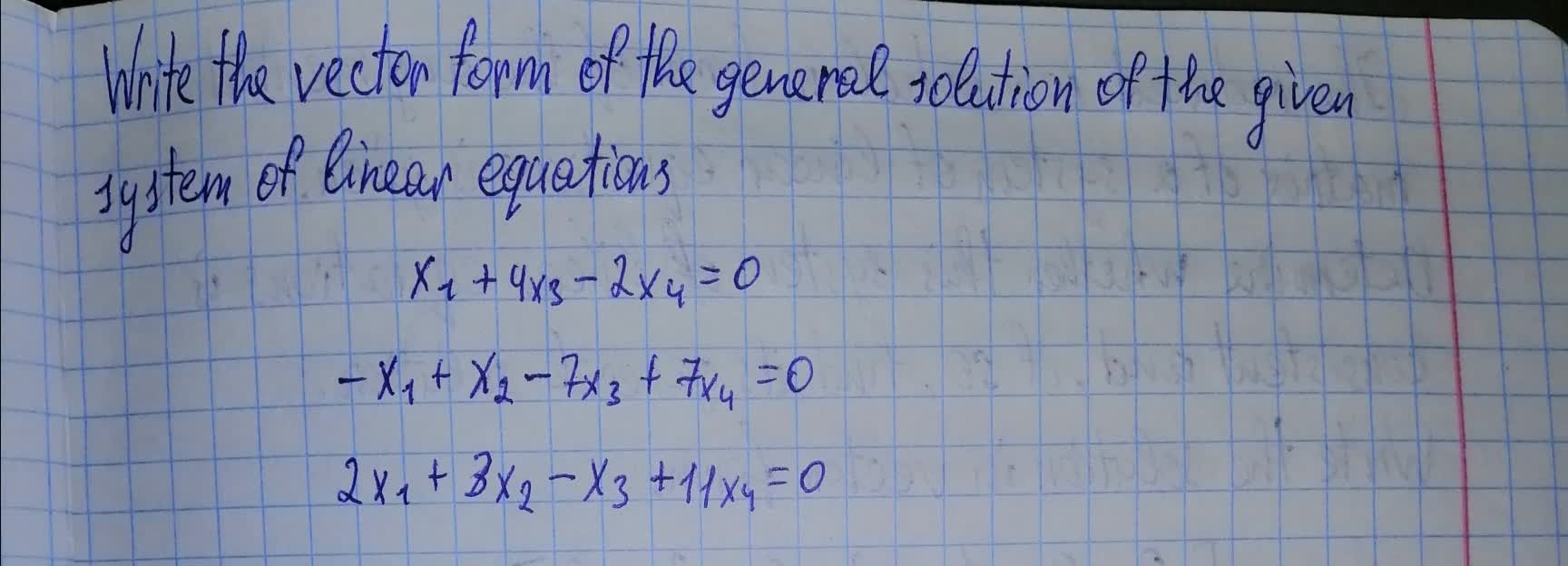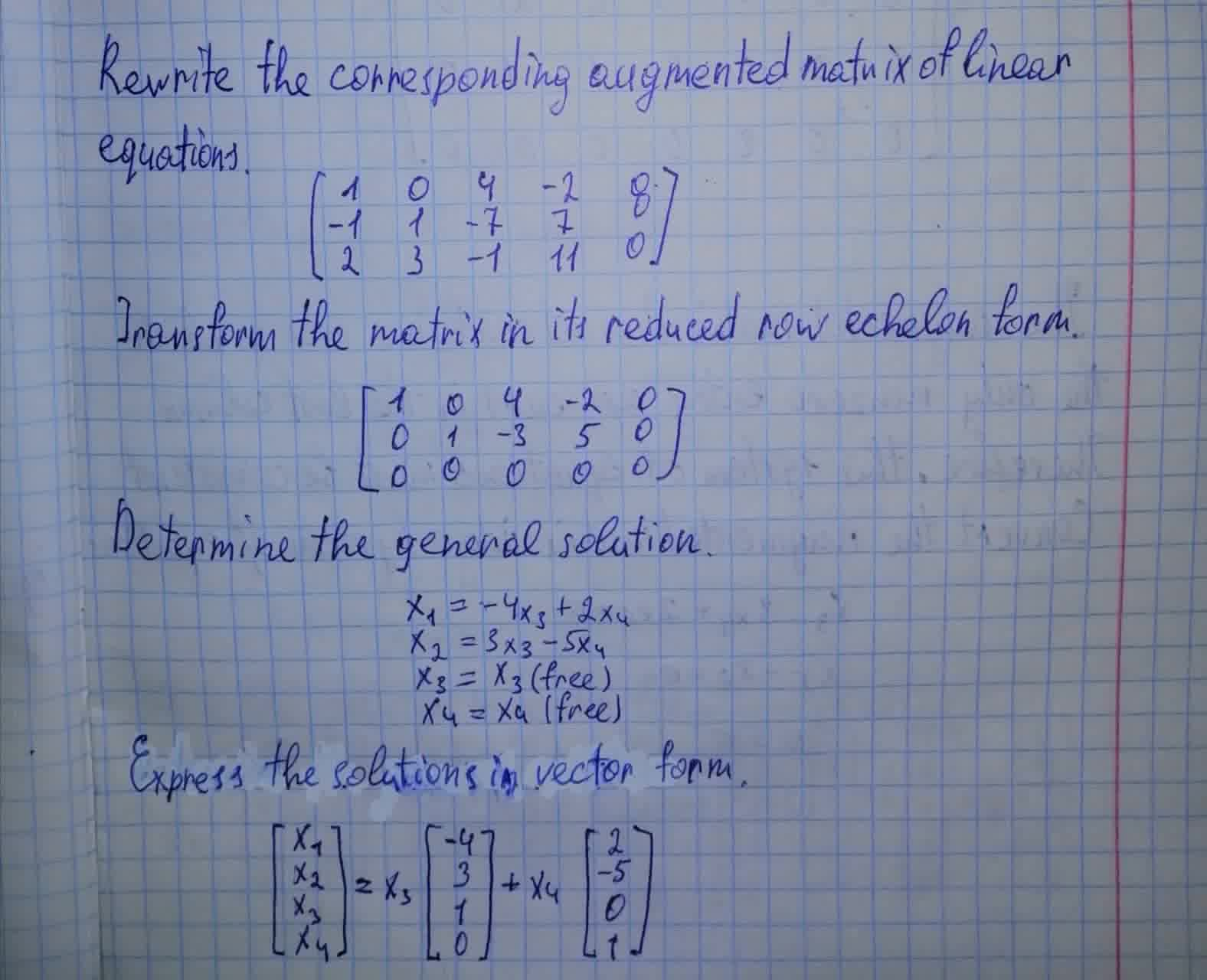# Write the vector form of the general solution of the given system of linear equations. x_1+4x_3-2x_4=0illusiia 2021-08-09 Answered
Write the vector form of the general solution of the given system of linear equations. ${x}_{1}+4{x}_{3}-2{x}_{4}=0$You can still ask an expert for help

• Questions are typically answered in as fast as 30 minutes

Solve your problem for the price of one coffee

• Math expert for every subject
• Pay only if we can solve itSoosteethicU
Answer is given in the photo below.###### Not exactly what you’re looking for?Vasquez

Rewrite the corresponding augmented matrix of the system of linear equation

$\left[\begin{array}{ccccc}1& 0& 4& -2& 0\\ -1& 1& -7& 7& 0\\ 2& 3& -1& 11& 0\end{array}\right]$

Transform the matrix in its reduced row echelon form

$\left[\begin{array}{ccccc}1& 0& 4& -2& 0\\ 0& 1& -3& 5& 0\\ 0& 0& 0& 0& 0\end{array}\right]$

Determine the general solution

${x}_{1}=-4{x}_{3}-2{x}_{4}$
${x}_{2}=3{x}_{3}-5{x}_{4}$
${x}_{3}={x}_{3}$ (free)
${x}_{4}={x}_{4}$ (free)

Express the solutions in vector form

$\left[\begin{array}{c}{x}_{1}\\ {x}_{2}\\ {x}_{3}\\ {x}_{4}\end{array}\right]={x}_{3}\left[\begin{array}{c}-4\\ 3\\ 1\\ 0\end{array}\right]+{x}_{4}\left[\begin{array}{c}2\\ -5\\ 0\\ 1\end{array}\right]$